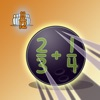# 4 Dice a Fractions Game - Lumos Educational App Store4.51
Price -Free
\$0

#### DESCRIPTION:

Multiplayer Math Game (up to 5 devices)! You can now use two devices and play competitively or cooperatively with your classmates or parents. 4 Dice: Fraction Games hones the many concepts of fractions that include adding, subtracting, multiplying and dividing through a series of game mode options. The game also enhances analytical thinking by providing the answer and requiring students to determine the appropriate equation. KEY FEATURES: - Multiplayer Math Game (up to 5 devices)! - High order thinking game to challenge students in a fun way - Progress reports sent to teachers to assess progre

#### OVERVIEW:

4 Dice a Fractions Game is a free educational mobile app By Justin Holladay.It helps students in grades 4 practice the following standards 4.NF.A.1,4.NF.A.2,4.NF.B.3.A.

This page not only allows students and teachers download 4 Dice a Fractions Game but also find engaging Sample Questions, Videos, Pins, Worksheets, Books related to the following topics.

1. 4.NF.A.1 : Explain why a fraction a/b is equivalent to a fraction (n × a)/(n × b) by using visual fraction models, with attention to how the number and size of the parts differ even though the two fractions themselves are the same size. Use this principle to recognize and generate equivalent fractions. (Grade 4 expectations in this domain are limited to fractions with denominators 2, 3, 4, 5, 6, 8, 10, 12, and 100.).

2. 4.NF.A.2 : Compare two fractions with different numerators and different denominators, e.g., by creating common denominators or numerators, or by comparing to a benchmark fraction such as 1/2. Recognize that comparisons are valid only when the two fractions refer to the same whole. Record the results of comparisons with symbols >, =, or <, and justify the conclusions, e.g., by using a visual fraction model. (Grade 4 expectations in this domain are limited to fractions with denominators 2, 3, 4, 5, 6, 8, 10, 12, and 100.).

3. 4.NF.B.3.A : Understand addition and subtraction of fractions as joining and separating parts referring to the same whole..

4

#### STANDARDS:

4.NF.A.1
4.NF.A.2
4.NF.B.3.A
4.NF.B.4
4.NF.C.6

Software Version: 2.1

Category: Education

### RELATED APPSEdSearch WebSearch